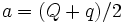# Semi-major axis

The semi-major axis of any ellipse is the axis of symmetry that passes through the foci of that ellipse.

In astrophysics, the semi-major axis of an orbit is the distance between the geometric center of the orbit and either apsis. It is a commonly reported orbital element that can determine the size and shape of an orbit.

## Calculations

The semi-major axis, usually labeled a, is the arithmetic mean of the two apsides:For this reason the semi-major axis is often cited as a "mean orbital radius."

The semi-major axis, together with the orbital eccentricity (e), can predict the periapsis and apoapsis:See orbital eccentricity for a detailed derviation